# Greens PHP Polygon Area: Calculate the area and perimeter of a polygon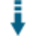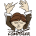Download .zip
 InfoExampleView files (6)Download .zip Reputation Support forum Blog Links
 Ratings Unique User Downloads Download Rankings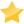47% Total: 290 All time: 7,481 This week: 148Version License PHP version Categories greens-polygon 1.0.0 GNU General Publi... 5 Algorithms, PHP 5, Geography, Math
Description

Author

This class can calculate the area and perimeter of a polygon.

It implements the Green's theorem to calculate the area of an irregular polygonal shape given the coordinates of the vertices.

The class can also calculate the perimeter of the polygon.

Innovation AwardSeptember 2015 Number 6Prize: One year server license IP to country, region, city, latitude, longitude, ZIP code, time zone, area code database Calculating the area of regions on the map is important for instance to determine the value of a land property. However, when the land shape is not rectangular, it is not trivial to compute the property area. This class can compute the area of irregular polygons that form regions on the map using the Greens theorem. Manuel LemosPerformance Level
Name: Classes: Samuel Adeshina is available for providing paid consulting. Contact Samuel Adeshina . 19 packages by Samuel Adeshina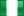Nigeria 24 527 2 in Nigeria39812 in Nigeria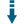Innovation awardNominee: 7x

Winner: 1x

## Recommendations

Area of polygon in Google Maps
Calculate the area of polygon drawn in a map

Area of a polygon
How to calculate the area of a polygon?

## Example

 ``` setVertices(new Point(0, 0), new Point(1.5, 0), new Point(2.5, -1), new Point(2, 5));     echo (\$polygon->area()); //returns the area of the irregular polygonal shape     echo (\$polygon->perimeter()); //returns the perimeter of the polygon (the distance around the polygonal shape or object) ?> ```

## Details

### How To Instantiate This Class

It determines the area and perimeter of a polygon by accepting the vertices (_coordinates_) of the polygon. _These parameters can be provided in three different ways_: 1. Supplying them in the constructor definition You can do this by adding the coordinates as a Point object as parameter.

A Point Object is a new instance of a point class that's already been included in the `polygon.class.php` class file. You can find the source code of this class in the `point.class.php` class file To provide the vertices of the polygon as parameters of the constructor, _you may instantiate it this way_:

```````\$polygonObjectInstance = new Polygon(new Point(0, 0), new Point(2), ...);`
``````

The constructor accepts as many parameters as is possible, _although, they're all optional_ 2. Providing them as parameters to the setVertice() method The setVertice method accepts a single parameter which must be an instance of the Point object. This parameter acts as the coordinate/vertex of a polygon

``````
`....`
`\$pointObjectInstance->setVertice(new Point());`
`\$pointObjectInstance->setVertice(new Point(2, 3));`
`//This option provides a coordinate with the x and y axis at the (0, 0) and (2, 3) positions on a plane`
``````
1. Providing them as parameters to the setVertices() method Unlike the setVertice method, this method accepts more than one parameter just like the constructor but they all do the same thing, to populate an array with a set of coordinates.

You can get both the area and parameter by calling either of the `area()` or `perimeter()` methods respectivelyFiles
File Role DescriptionHow-To.md Doc. A quick tutorialLICENSE Data License Filepoint.class.php Class Point Classpolygon.class.php Class Polygon ClassREADME.md Data Read Metest.php Example Examples And How To

 All time: 7,481 This week: 148All time Utility: 58%58%66%58%- - 47%2594About usAdvertise on this siteSite mapNewsletterStatisticsSite tipsPrivacy policyContact
For more information send a message to `info at phpclasses dot org`.